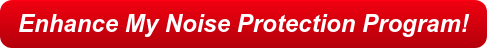Jeff Griggs - Feb 18, 2019

# We work 12-hour shifts. What dosimeter criterion time should we use?

Is it just me, or can trying to decipher an OSHA regulation be confusing? Take, for instance, the OSHA Occupational Noise Exposure Standard (29 CFR 1910.95). The OSHA noise standard defines the action level to enroll an employee in a hearing conversation program as “an 8-hour time-weighted average of 85 decibels measured on the A-scale, slow response, or equivalently, a dose of 50 percent.” Because the action level is based on an exposure for an 8-hour work shift, a common point of confusion is what to do for work shifts that are not 8 hours, such as 10- or 12-hour work shifts. A common question we are asked is, “We work a 12-hour shift: Should I use 8 or 12 as the time duration on my noise dosimeter to calculate the time-weighted average?” Understanding how changing the time duration of a noise dosimeter sample affects the results, and how this relates to the noise standard, will help us make the best decision.

The OSHA-defined action level is a reference point for the amount of noise energy someone may be exposed to. It contains three primary values that make up the reference point: The 8-hour time period, the 85 dB noise level, and the 50 percent dose. If we change one of these elements, there has to be a corresponding change to a second element for the third element to remain true. Therefore, if the time period increases to 12 hours, there must be a decrease in the average noise level for the dose to remain at 50 percent.

The criterion time is the time duration reference that is used in the calculation of the TWA. If the amount of time spent in a noise environment doubles, and the noise level remains the same, then the exposure dose will also be doubled. If the time spent is reduced by half, then the exposure will be halved.

Using a 12-hour criterion time for a 12-hour shift requires that the reference noise level be lowered from 85 dB to 82.5 dB for the dose to remain at 50 percent. What this means to you is that if you change the criterion time to 12 hours, you must remember to make your decisions based on a TWA of 82.5 dB to remain compliant.

Using an 8-hour criterion time for a 12-hour shift requires that you use a reference noise level of 85 dB as listed in the OSHA standard. The reason for this is that the instrument does all the work. The dosimeter takes the noise energy measured over the 12 hours and calculates it back to the OSHA reference point. Your outcome is simplified in that there is no need to remember to make adjustments to the TWA for compliance decisions. Also, you will not have to explain why changes were made to the standard settings.

Let OHD help bring your Sound Monitoring  Program  up to par in 2019!